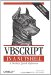# Section A.10. Information Functions

### A 10 Information Functions

 GetLocale Function Returns the ID of the current locale IsArray Function Returns True if a variable is an array IsDate Function Returns True if an expression can be converted to a date IsEmpty Function Returns True if a variant variable has not been initialized IsNull Function Returns True if an expression evaluates to Null IsNumeric Function Returns True if an expression can be evaluated as a number IsObject Function Returns True if a variable contains an object reference Len Function Returns the length of a variable LenB Function Returns the number of bytes needed to hold a given variable RGB Function Returns a number representing an RGB color value ScriptEngine Function Returns a string representing the scripting language in use ScriptEngineBuildVersion Function Returns VBScript's build number ScriptEngineMajorVersion Function Returns VBScript's major version number ScriptEngineMinorVersion Function Returns VBScript's minor version number TypeName Function Returns the data type name of a variable VarType Function Returns a number representing the data type of a variableVBScript in a Nutshell, 2nd Edition
ISBN: 0596004885
EAN: 2147483647
Year: 2003
Pages: 335